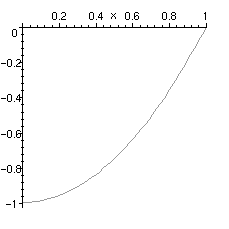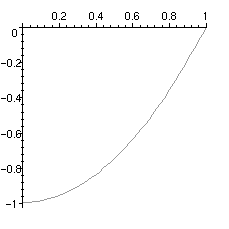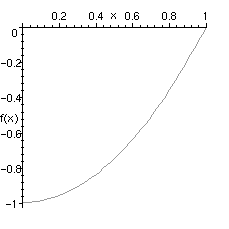Usually one plots expressions in Maple, for example

> plot(x^2-1, x = 0 .. 1);Note that the independent variable is used in specifying the interval on which to plot. If you left this out, Maple would not know what variable to use, resulting in an error:

> plot(x^2-1, 0 .. 1);

Plotting error, empty plot

It is also possible to plot functions rather than expressions. This is particularly useful with functions that are meant to be called only with numerical, rather than symbolic, arguments.

> f:= x -> x^2-1:

> plot(f, 0 .. 1);In this case you do not specify the name of the variable. If you did, you would get strange-looking results:

> plot(f, x = 0 .. 1);If you wish to label the axes in such a plot, you should use the labels option.

> plot(f, 0 .. 1, labels=[x,`f(x)`]);Maple Advisor Database R. Israel 1997